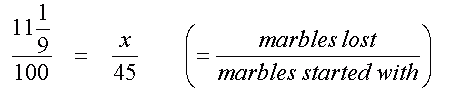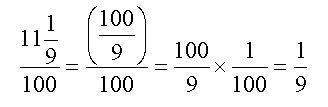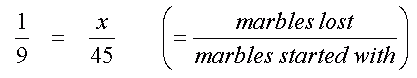SEARCH HOMEMath Central Quandaries & QueriesIf Jim had 45 marbles and he lost 11 1/9% of them, how many had he left?We have two responses for you.

Hi Mesha,

11 1/9% = 100/9 %

Remember that % mean per hundred, so that is (100/9) / 100 which
equals simply 1/9.

So what is 1/9 of 45? That's how many marbles Jim lost.

Stephen La Rocque.>

Hi Mesha,

% means 'out of a hundred', so the question tells you that he lost 11 1/9 out of hundred marbles. Of course, he didn't really have 100 marbles, and you can't really lose 1/9 of a marble (unless you smash the marble), but this is very useful information because it tells you the ratio of the number of marbles Jim lost compared to the number of marbles he started with. So, we can set up a relationship to help find the number of marbles Jim actually lost:

Let x be the number of marbles Jim lost (the number we are trying to find).Next we can simplify the ratio on the left to make it easier to see what x has to be. I'll convert the mixed fraction to an improper fraction (which is more proper for what we need to do), and then reduce.So we can write the first expression like this:Can you see what x has to be to make these two ratios the same? What part of 45 is one ninth?

Hope this helps,
HaleyMath Central is supported by the University of Regina and The Pacific Institute for the Mathematical Sciences.